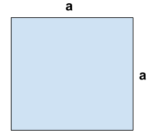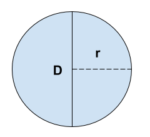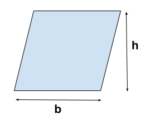# Basic Surface Area and Circumference Formulas

This may be funny or weird if you are new to the field. Even experienced guys also sometimes surf the internet to find these basic formulas.

You don’t need to memorize, the more you use this formula in day to day work the more it will be automatically stored in your mind.

Believe me, guys, this will be useful forever in your civil life.

 NAME FIGURE AREA PERIMETER CIRCUMFERRENCE SquareA = a2 P = a + a + a + a = 4a TriangleA = (b x h) / 2 P = AB + BC + CA CircleA= π r2 C = 2πr = πD TrapezoidA = ((b1 + b2) x h) / 2 P = AB + BC + CD + DA ParallelogramA = b x h P = AB + BC + CD + DA RectangleA = L x b P = L + b + L + b P = 2L + 2b HexagonA = (3√3/ 2) a2 P = 6a